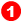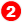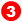﻿ Menu | Function-Block | Gearing

# Function-Block | Gearing

### Why use a Gearing Function-Block?

 The Gearing FB applies a simple linear equation to motion-values at its input-connector. The linear equation: Output motion-value = (* (Input motion-value +)) +After you add a Gearing FB, open the Gearing FB dialog-box to edit the parameters in the linear equation.

### Gearing FBKinematics FB toolbar > Gearing FB [right of Graphic-Area >>]

STEP 1: Add the Gearing FB to the graphic-area

 1 Click Kinematics FB Toolbar  > Gearing FB
 2 Click again in the graphic-area

The Gearing FB is now in the graphic-area.

To Edit:

STEP 2: Open the Gearing FB dialog-box:

 1 Double-click a Gearing FB in the graphic-area

- or -

 1 .#### GEARING FB DIALOG-BOX

Ratio and Phase

Gearing Ratio ['Real' #]

Enter the Gearing Ratio parameter in one of two ways. You can enter it asIrrational Ratio or a Rational Ratio [see below]

Add after Gear RatioThe FB adds this number after the Gearing Ratio parameter

Add before Gear RatioThe FB adds this number before the Gearing Ratio parameter

Gearing as 'Real' or 'Ratio: 'Real' or 'Ratio'

 ◉ Gearing as 'Real : Use the Gearing Ratio: - see above◉ Gearing as 'Ratio'Use the 'Input Pulley and 'Output Pulley' parameters.

Enter positive or negative values for the 'Input Pulley' and the 'Output Pulley' parameters to give the Gearing Ratio

The ratio of the two numbers is a 'rational' number.

Notes:

The default parameter values do not change the motion-values at the output-connector.

 • Use a Gearing Ratio of '-1' to reverse the direction of a Crank.
 • Connect a Gearing FB to the input of a Motion FB to change the frequency of the motion.
 • Connect a Motion FB to the input of a Gearing FB to change the amplitude or direction, or both, of the motion.